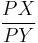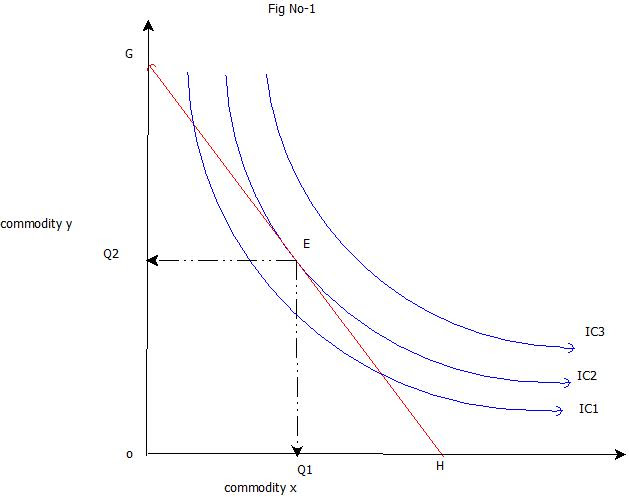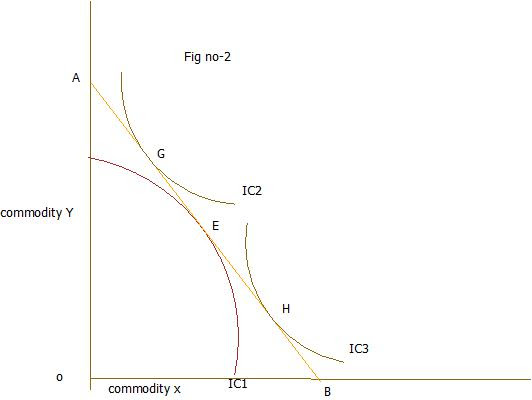### Ticker

6/recent/ticker-posts

# budget line and consumer equilibrium

### Budget Line

The budget line shows all the combinations of two goods that can be purchased with a given level of income.Let the blue line in the figure above be the consumer's original budget line. If the consumer's income increases, the budget line would shift out, say, to the pink line. A change in the price of one of the goods, on the other hand, rotates the budget line along the axis of the good experiencing the change. A fall in price rotates the budget line out. An increase in the price of the good on the X-axis would rotate the budget line in, for example, to the green line.

in other words
Just as production possibilities frontiers limit society s ability to produce and consume, each individual family is constrained by its income in choosing among consumption alternatives. A consumer s budget line is usually straight because the purchasing pattern a family chooses seldom affects market prices.
A budget line (or constraint) depicts the choices available to a consumer who faces constant prices and who has a given income.
Figure 5 shows budget lines for two levels of income using the assumption that asparagus (A) and a proxy for all other goods, called shmoo (B), each cost $1 per pound. Any point in the AB space represents a specific combination of asparagus and shmoo and would require a certain level of income.We can write the family s budget constraint as equal towhere Pa and Pb are the prices of asparagus and shmoo, respectively, and all family income (Y) is spent on either asparagus (A) or shmoo (B). Because income is limited, asparagus can be traded for shmoos as long as the family stays within its income constraint. Subtracting PaA from each side yieldsFinally, we can solve for the amounts of B, given certain purchases of A, if we divide this equation by Pb:This equation defines the budget line for the family in Figure 5. Because both asparagus and shmoo cost$1 per pound (Pa = Pb), point v represents the situation where the family has $600 per week, all of which is spent on shmoo. Note that Y/Pb =$600/$1 = 600 pounds of shmoo. Point z represents just the opposite: all income is spent on asparagus, nothing is spent on shmoo. The slope of the budget line equals  Pa/Pb, which equals  1, because shmoo and asparagus are priced identically. Thus, as income changes, the family s budget line moves in or out, but as long as the ratio of the two prices remains the same, the slope of the budget line will not change. The budget line pivots if asparagus prices double to$2 a pound. A consumer with a $600 weekly income could now buy a maximum of only 300 pounds of asparagus, but could still buy as much as 600 pounds of shmoo. Alternatively, if the price of asparagus fell to$0.50 a pound, up to 1,200 pounds of asparagus might be purchased from a $600 income. Budget lines reflecting various possible prices for asparagus, with the price of shmoo still at$1 a pound and with a \$600 weekly budget, are shown in Figure 6.### Consumer Equilibrium

The objective of consumers is to be on the highest possible indifference curve. The budget line shows all the bundles the consumer can afford. So, the best bundle the consumer can afford is found where the budget line is tangent to the highest possible indifference curve. In the diagram below, consumer equilibrium occurs at point E.At the consumer's equilibrium, the amount of beer the consumer is willing to give up in exchange for one more slice of pizza is equal to the amount of beer the market requires her to give up in order to obtain one more slice of income, everything else the same.

in other words,
All consumers strive to maximize their utility. We try to get as much satisfaction as we can. The consumer’s scale of preference is derived by means of indifference mapping that is a set of indifference curves which ranks the preferences of the consumer. Getting to the indifference curve which is farthest from the origin gives the highest total utility. Although the goal of the consumer is maximization of satisfaction, the means of achieving the goal is not clear. Higher indifference curve not only gives higher satisfaction but also are more expensive. Here we are confronted with the basic conflict between preferences and the prices of the commodities consumer wants to consume. With a given amount of money income to spent, we cannot attain the highest satisfaction but have to settle for less.
Assumptions

.
• 1.There are two goods i.e commodity X and commodity Y .
• 2.The consumer’s preference scale for combination of two goods is exhibited by indifference map.
• 3.The prices of goods are given and remain constant.
• 4.The consumer has a given income which sets to limits to his maximizing behavior.

Conditions for consumer's equilibrium
• 1.A given budget line must be tangent to an indifference curve , or the marginal rate of substitution between commodity X and commodity Y (MRSx,y) must be equal to the price ratio between the two goods.
• 2.At the point of equilibrium, indifference curve must be convex to the origin.

The limitation on utility maximization is evident. We want to reach the highest indifference curve with our limited income. You can go only as far as your budget constraint allows. Suppose you have only 50 rupees to spend on good X and good Y. The price of a unit of X is 10 rupees where as the price of good Y is 5 rupees. You can have as many as 5 units of good X if you want to forsake good Y. Similarly you can have 10 units of good Y with the same 50 rupees.The budget constraints illustrates all combination of goods you can buy with a limited income. In this case the budget line illustrates the combination of X and Y , that can be purchased with 50 rupees.Above diagram explain the process of consumer’s equilibrium . The consumer’s preference scale is described by means of indifference mapping .Then we impose a budget line that reflects our income. In this case we have r 50 and the price of good X and good Y is r 10 and r 5 respectively. Therefore, we can afford only those combinations that are on or inside the price line GH.

In this diagram every combination on the price line GH cost you the same amount of money. In order to maximize the utility , we will try to reach the highest indifference curve which you could get with a given expenditure of money and given prices of two goods.The budget line touches IC2 at point E represents the most utility. This is the highest attainable indifference curve with which you can get OQ1 units of good X and OQ2 units of good Y for r 50. Any other affordable combinations on the price line GH gives you less satisfaction, because that will be on a lower indifference curve IC1. With this we conclude that the point of tangency between the budget line and an indifference curve represents optimal consumption. It is the affordable combination that maximize our utility.

At the tangency point E the slope of the price line GH and indifference curve are equal. Slope of the indifference curve shows the marginal rate of substitution of X for Y. The price line indicates the ratio between the prices of two goods (PX/PY). Thus at the equilibrium point E,MRSXY=Price of good x/Price of good y= PX/PY

The tangency between the given price line and an indifference curve is a necessary but not a sufficient condition consumer’s equilibrium .The second condition for consumer’s equilibrium is convexity of indifference curve to the origin .Which means MRSxy is falling at the point of equilibrium.In fig no -1 indifference curve IC2 is convex to the origin at point E, is the optimum or best choice for the consumer .The consumer attains a stable equilibrium position where he is able to consume the most preferred combination which gives him highest utility. In figure no -2 ,IC1 is concave to the origin at point E. Price line AB is tangent to the indifference curve IC1 at point E and the marginal rate of substitution of X for Y is equal to the price ratio of two goods (PX/PY). But E cannot be the position of stable equilibrium because satisfaction would not be maximum .There are other combinations like G and H in the given price line will be on higher indifference curve .The congumer by moving along the given price line AB can go to other tangency pointsuch as G and H and obtain greater satisfaction than at point E.